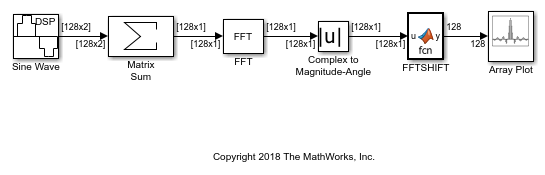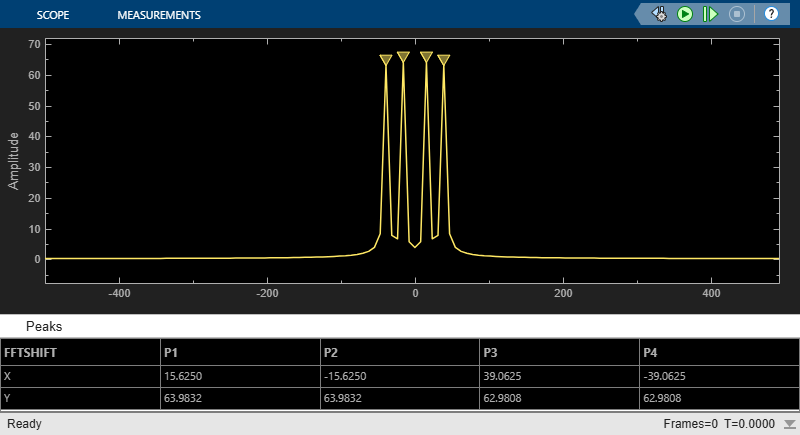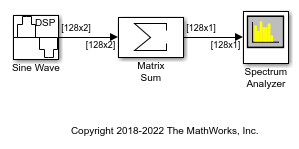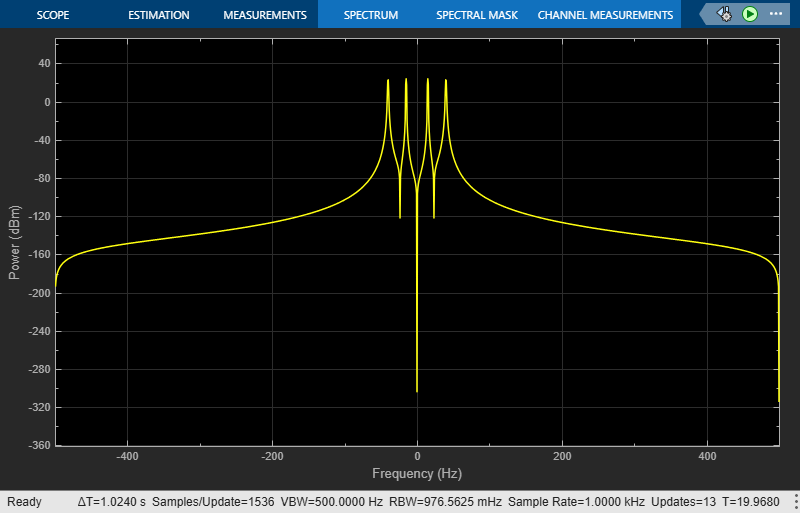Documentation

## Transform Time-Domain Data into Frequency Domain

This example shows how to transform time-domain data into the frequency domain using the FFT block.

Use the Sine Wave block to generate two sinusoids, one at 15 Hz and the other at 40 Hz. Use the Matrix Sum block to add the sinusoids point-by-point to generate the compound sinusoid:

``

Transform this sinusoid into the frequency domain using an FFT block. See the `ex_fft_tut` model:The scope shows peaks at 15 and 40 Hz, as expected. You have now transformed two sinusoidal signals from the time domain to the frequency domain.

You can use a Spectrum Analyzer block in place of the sequence of FFT, Complex to Magnitude-Angle, MATLAB Function, and Array Plot blocks. The Spectrum Analyzer computes the magnitude FFT and shifts the FFT internally. See the `ex_time_freq_sa` model:The blocks in the Power Spectrum Estimation library compute the FFT internally.

Watch now